## R语言游戏之旅 贪食蛇入门

R的极客理想系列文章，涵盖了R的思想，使用，工具，创新等的一系列要点，以我个人的学习和体验去诠释R的强大。

R语言作为统计学一门语言，一直在小众领域闪耀着光芒。直到大数据的爆发，R语言变成了一门炙手可热的数据分析的利器。随着越来越多的工程背景的人的加入，R语言的社区在迅速扩大成长。现在已不仅仅是统计领域，教育，银行，电商，互联网….都在使用R语言。

• 张丹(Conan), 程序员Java,R,PHP,Javascript
• weibo：@Conan_Z
• blog: http://blog.fens.me
• email: bsspirit@gmail.com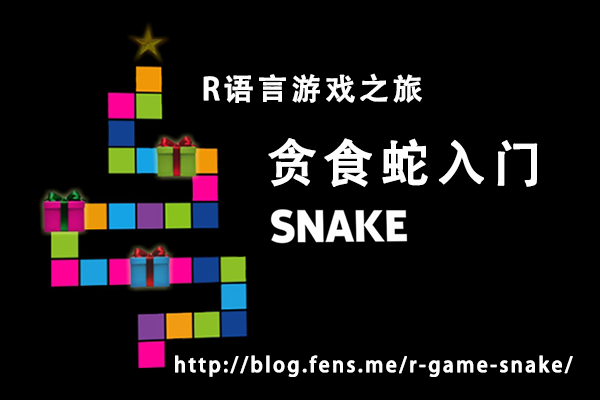1. 贪食蛇游戏介绍
2. 场景设计
3. 程序设计
4. R语言实现

## 1. 贪食蛇游戏介绍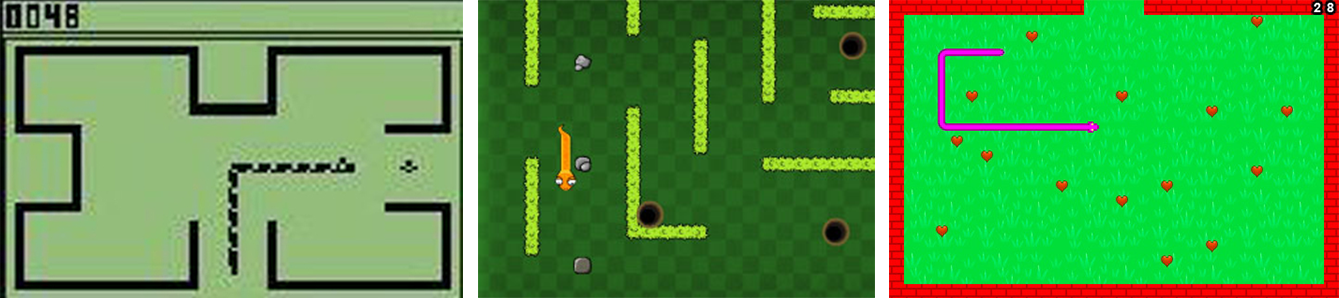## 2. 场景设计

2.1 需求分析

• 开机场景：运行程序，在游戏前，给用户做准备，并提示如何操作游戏。
• 游戏场景：游戏运行中的场景。
• 结束场景：当用户胜利、失败或退出时的场景，并提示用户在游戏中的得分。

2.2 游戏规则

• 1. 开始游戏后，用户可以通过上(up)下(down)左(left)右(right)键，来操作蛇头，控制蛇的前进方向，还可以按q键直接游戏失败，其他的键盘操作无效。
• 2. 蛇头用蓝色标识，蛇尾用灰色标识，水果用红色标识，障碍物用黑色标识。
• 3. 当蛇头移动到水果的位置后，表示蛇吃到了水果，蛇尾的长度加1。水果会在下一次蛇头移动后，在空路径上自动生成。
• 4. 游戏画布的外围是枪，当蛇头移动到画布看不到的位置，则表示蛇头撞到枪，游戏失败。
• 5. 游戏画面中，有一些黑色障碍物，当蛇头碰到障碍，游戏失败。
• 6. 当蛇头碰到蛇尾时，游戏失败。

2.3 业务流程

• 打开程序时，用户首先看到开机场景，按任意键后进入游戏场景。
• 在游戏场景，当游戏失败，进入结束场景；按q键，则直接游戏失败。
• 在结束场景，按空格回到开机场景；按q键，则直接能出软件。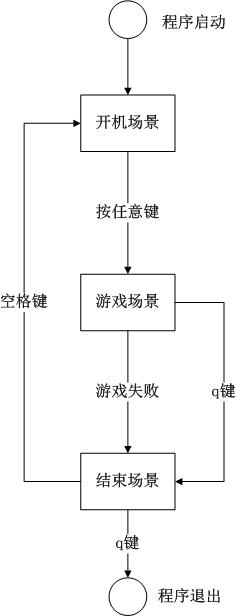2.4 游戏原型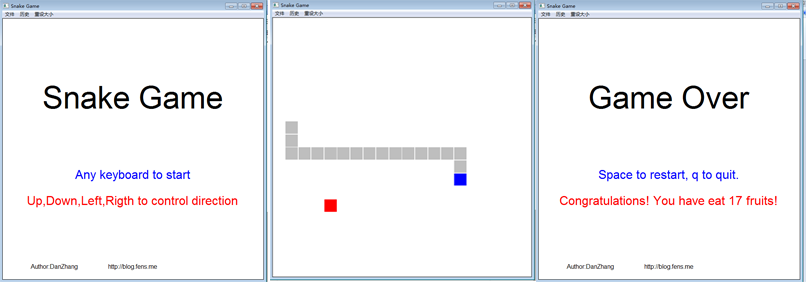## 3. 程序设计

3.1 游戏场景

• 开机场景，是静态的，我们可以提前生成好这块画布存储起来，也可以当用户切换时再临时生成，性能开销不大。
• 游戏场景，是动态的，每进行一次用户的交互行为或按时间刷新时，都需要求重新绘制画布，让游戏场景通过绑定事件来生成画布。由于用户会频繁操作，因此性能开销比较大。
• 结束场景，是动态的，在结束场景会显示当次游戏的得分，需要在切换时临时生成。

3.2 游戏对象

• 画布：用矩阵来描述，画布中每个小方块对应到矩阵中一个数据。
• 画布大小：画布的长和宽，分别用对应两个数字变量。
• 画布坐标：用于画布内小格子的定位，从左到右横坐标是1到20，从底到顶纵坐标为1到20。
• 画布索引：用于画布内小格式的定位，按从左到右，从底到顶的顺序，为1到400。
• 方格：在画布里最小的单位是方格，按照画面的比例，设置方格的大小。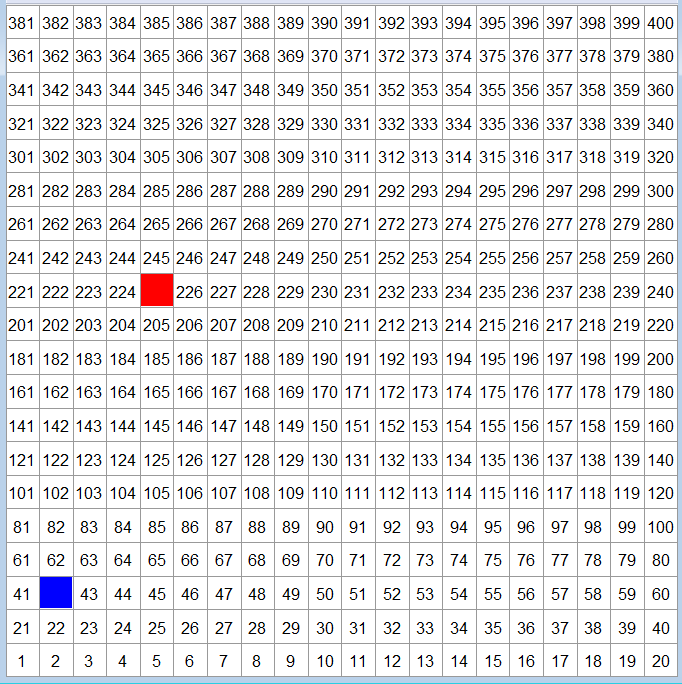• 蛇头：用一个向量来描述，只有一个方格。游戏开始时，起点位置为坐标(2,2)，默认蛇头向上移动，用户打开界面显示位置为(2,3)。
• 蛇尾：用数据框来描述，存储不定长度的坐标向量。游戏开始时，蛇尾长度是0。

• 水果：用一个向量来描述，只有一个方格。游戏开始时，随机在空格式上，选一个坐标为水果位置。

• 边界：无内存描述，通过计算判断。当蛇头坐标超过矩阵坐标时，触发边界。
• 障碍物：用数据框来描述，存储不定长度的坐标向量。

3.3 游戏事件

• 键盘事件：全局事件，用户通过键盘输入，而触发的事件，比如，上下左右控制蛇的移动方向。
• 时间事件：全局事件，系统计时以每0.2秒触发一个时间事件，比如，蛇头每0.2秒的移动一格。
• 碰撞事件：当蛇头移动时，与非空和格式碰撞除法的事情，比如，吃到水果，蛇头撞到蛇尾。

3.4 游戏控制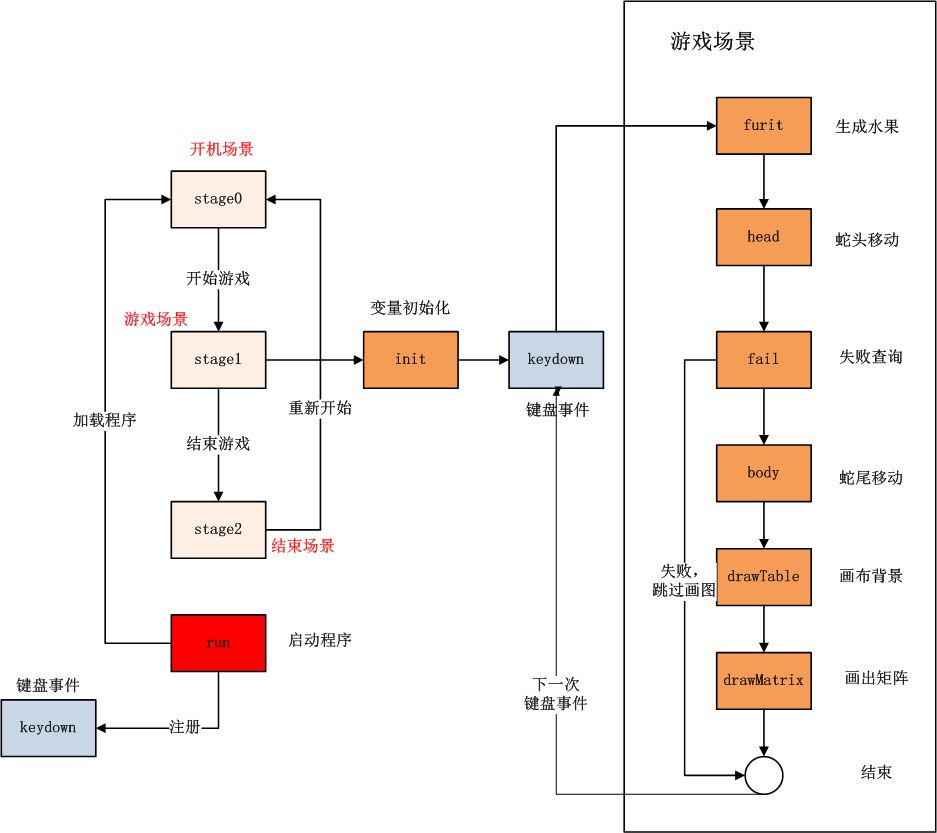• run():启动程序。
• keydown():监听键盘事件，锁定线程。
• stage0():创建开机场景，可视化输出。
• stage1():创建游戏场景，可视化输出。
• stage2():创建结束场景，可视化输出。
• init():打开游戏场景时，初始化游戏变量。
• furit():判断并生成水果坐标。
• fail():失败查询，判断蛇头是否撞墙或蛇尾，如果失败则跳过画图，进入结束场景。
• body():生成蛇尾移动坐标。
• drawTable():绘制游戏背景。
• drawMatrix():绘制游戏矩阵。

## 4. R语言实现

run()函数，启动程序。

``````
run<-function(){
# 设置全局画布无边
par(mai=rep(0,4),oma=rep(0,4))

# 定义全局环境空间，用于封装变量
e<<-new.env()

# 启动开机场景
stage0()

# 注册键盘事件
getGraphicsEvent(prompt="Snake Game",onKeybd=keydown)
}
``````

keydown函数，监听键盘事件。

``````
keydown<-function(K){
print(paste("keydown:",K,",stage:",e\$stage));

if(e\$stage==0){ #开机画面
init()
stage1()
return(NULL)
}

if(e\$stage==2){ #结束画面
if(K=="q") q()
else if(K==' ') stage0()
return(NULL)
}

if(e\$stage==1){ #游戏中
if(K == "q") {
stage2()
} else {
if(tolower(K) %in% c("up","down","left","right")){
e\$lastd<-e\$dir
e\$dir<-tolower(K)
stage1()
}
}
}
return(NULL)
}
``````

stage0():创建开机场景，可视化输出。

``````
# 开机画图
stage0<-function(){
e\$stage<-0
plot(0,0,xlim=c(0,1),ylim=c(0,1),type='n',xaxs="i", yaxs="i")
text(0.5,0.7,label="Snake Game",cex=5)
text(0.5,0.4,label="Any keyboard to start",cex=2,col=4)
text(0.5,0.3,label="Up,Down,Left,Rigth to control direction",cex=2,col=2)
text(0.2,0.05,label="Author:DanZhang",cex=1)
text(0.5,0.05,label="http://blog.fens.me",cex=1)
}
``````

stage2():创建结束场景，可视化输出。

``````
# 结束画图
stage2<-function(){
e\$stage<-2
plot(0,0,xlim=c(0,1),ylim=c(0,1),type='n',xaxs="i", yaxs="i")
text(0.5,0.7,label="Game Over",cex=5)
text(0.5,0.4,label="Space to restart, q to quit.",cex=2,col=4)
text(0.5,0.3,label=paste("Congratulations! You have eat",nrow(e\$tail),"fruits!"),cex=2,col=2)
text(0.2,0.05,label="Author:DanZhang",cex=1)
text(0.5,0.05,label="http://blog.fens.me",cex=1)
}
``````

init():打开游戏场景时，初始化游戏变量。

``````
# 初始化环境变量
init<-function(){
e<<-new.env()
e\$stage<-0 #场景
e\$width<-e\$height<-20  #切分格子
e\$step<-1/e\$width #步长
e\$m<-matrix(rep(0,e\$width*e\$height),nrow=e\$width)  #点矩阵
e\$dir<-e\$lastd<-'up' # 移动方向
e\$lastx<-e\$lasty<-2 # 初始化蛇头上一个点
e\$tail<-data.frame(x=c(),y=c())#初始蛇尾

e\$col_furit<-2 #水果颜色
e\$col_tail<-8 #蛇尾颜色
e\$col_path<-0 #路颜色
}
``````

furit():判断并生成水果坐标。

``````
# 随机的水果点
furit<-function(){
if(length(index(e\$col_furit))<=0){ #不存在水果
idx<-sample(index(e\$col_path),1)

fx<-ifelse(idx%%e\$width==0,10,idx%%e\$width)
fy<-ceiling(idx/e\$height)
e\$m[fx,fy]<-e\$col_furit

print(paste("furit idx",idx))
print(paste("furit axis:",fx,fy))
}
}
``````

fail():失败查询，判断蛇头是否撞墙或蛇尾，如果失败则跳过画图，进入结束场景。

``````
# 检查失败
fail<-function(){
print("game over: Out of ledge.")
keydown('q')
return(TRUE)
}

keydown('q')
return(TRUE)
}

return(FALSE)
}
``````

``````

# 方向操作

}
``````

body():生成蛇尾移动坐标。

``````
# snake body
body<-function(){
e\$m[e\$lastx,e\$lasty]<-0
if(length(index(e\$col_furit))<=0){ #不存在水果
e\$tail<-rbind(e\$tail,data.frame(x=e\$lastx,y=e\$lasty))
}

if(nrow(e\$tail)>0) { #如果有尾巴
e\$tail<-rbind(e\$tail,data.frame(x=e\$lastx,y=e\$lasty))
e\$m[e\$tail[1,]\$x,e\$tail[1,]\$y]<-e\$col_path
e\$tail<-e\$tail[-1,]
e\$m[e\$lastx,e\$lasty]<-e\$col_tail
}

}
``````

drawTable():绘制游戏背景。

``````
# 画布背景
drawTable<-function(){
plot(0,0,xlim=c(0,1),ylim=c(0,1),type='n',xaxs="i", yaxs="i")

# 显示背景表格
abline(h=seq(0,1,e\$step),col="gray60") # 水平线
abline(v=seq(0,1,e\$step),col="gray60") # 垂直线
# 显示矩阵
df<-data.frame(x=rep(seq(0,0.95,e\$step),e\$width),y=rep(seq(0,0.95,e\$step),each=e\$height),lab=seq(1,e\$width*e\$height))
text(df\$x+e\$step/2,df\$y+e\$step/2,label=df\$lab)
}
``````

drawMatrix():绘制游戏矩阵。

``````
# 根据矩阵画数据
drawMatrix<-function(){
idx<-which(e\$m>0)
px<- (ifelse(idx%%e\$width==0,e\$width,idx%%e\$width)-1)/e\$width+e\$step/2
py<- (ceiling(idx/e\$height)-1)/e\$height+e\$step/2
pxy<-data.frame(x=px,y=py,col=e\$m[idx])
points(pxy\$x,pxy\$y,col=pxy\$col,pch=15,cex=4.4)
}
``````

stage1():创建游戏场景，stage1()函数内部，封装了游戏场景运行时的函数，并进行调用。

``````
# 游戏中
stage1<-function(){
e\$stage<-1
furit<-function(){...} //见furit
fail<-function(){...} //见fail
body<-function(){...}//见body
drawTable<-function(){...} //见drawTable
drawMatrix<-function(){...} //见drawMatrix

# 运行函数
furit()
if(!fail()){ #失败检查
body()
drawTable()
drawMatrix()
}
}
``````

``````
# 初始化环境变量
init<-function(){
e<<-new.env()
e\$stage<-0 #场景
e\$width<-e\$height<-20  #切分格子
e\$step<-1/e\$width #步长
e\$m<-matrix(rep(0,e\$width*e\$height),nrow=e\$width)  #点矩阵
e\$dir<-e\$lastd<-'up' # 移动方向
e\$lastx<-e\$lasty<-2 # 初始化蛇头上一个点
e\$tail<-data.frame(x=c(),y=c())#初始蛇尾

e\$col_furit<-2 #水果颜色
e\$col_tail<-8 #蛇尾颜色
e\$col_path<-0 #路颜色
}

# 获得矩阵的索引值
index<-function(col) which(e\$m==col)

# 游戏中
stage1<-function(){
e\$stage<-1

# 随机的水果点
furit<-function(){
if(length(index(e\$col_furit))<=0){ #不存在水果
idx<-sample(index(e\$col_path),1)

fx<-ifelse(idx%%e\$width==0,10,idx%%e\$width)
fy<-ceiling(idx/e\$height)
e\$m[fx,fy]<-e\$col_furit

print(paste("furit idx",idx))
print(paste("furit axis:",fx,fy))
}
}

# 检查失败
fail<-function(){
print("game over: Out of ledge.")
keydown('q')
return(TRUE)
}

keydown('q')
return(TRUE)
}

return(FALSE)
}

# 方向操作

}

# snake body
body<-function(){
e\$m[e\$lastx,e\$lasty]<-0
if(length(index(e\$col_furit))<=0){ #不存在水果
e\$tail<-rbind(e\$tail,data.frame(x=e\$lastx,y=e\$lasty))
}

if(nrow(e\$tail)>0) { #如果有尾巴
e\$tail<-rbind(e\$tail,data.frame(x=e\$lastx,y=e\$lasty))
e\$m[e\$tail[1,]\$x,e\$tail[1,]\$y]<-e\$col_path
e\$tail<-e\$tail[-1,]
e\$m[e\$lastx,e\$lasty]<-e\$col_tail
}

}

# 画布背景
drawTable<-function(){
plot(0,0,xlim=c(0,1),ylim=c(0,1),type='n',xaxs="i", yaxs="i")
}

# 根据矩阵画数据
drawMatrix<-function(){
idx<-which(e\$m>0)
px<- (ifelse(idx%%e\$width==0,e\$width,idx%%e\$width)-1)/e\$width+e\$step/2
py<- (ceiling(idx/e\$height)-1)/e\$height+e\$step/2
pxy<-data.frame(x=px,y=py,col=e\$m[idx])
points(pxy\$x,pxy\$y,col=pxy\$col,pch=15,cex=4.4)
}

furit()
if(!fail()){
body()
drawTable()
drawMatrix()
}
}

# 开机画图
stage0<-function(){
e\$stage<-0
plot(0,0,xlim=c(0,1),ylim=c(0,1),type='n',xaxs="i", yaxs="i")
text(0.5,0.7,label="Snake Game",cex=5)
text(0.5,0.4,label="Any keyboard to start",cex=2,col=4)
text(0.5,0.3,label="Up,Down,Left,Rigth to control direction",cex=2,col=2)
text(0.2,0.05,label="Author:DanZhang",cex=1)
text(0.5,0.05,label="http://blog.fens.me",cex=1)
}

# 结束画图
stage2<-function(){
e\$stage<-2
plot(0,0,xlim=c(0,1),ylim=c(0,1),type='n',xaxs="i", yaxs="i")
text(0.5,0.7,label="Game Over",cex=5)
text(0.5,0.4,label="Space to restart, q to quit.",cex=2,col=4)
text(0.5,0.3,label=paste("Congratulations! You have eat",nrow(e\$tail),"fruits!"),cex=2,col=2)
text(0.2,0.05,label="Author:DanZhang",cex=1)
text(0.5,0.05,label="http://blog.fens.me",cex=1)
}

# 键盘事件
keydown<-function(K){
print(paste("keydown:",K,",stage:",e\$stage));

if(e\$stage==0){ #开机画面
init()
stage1()
return(NULL)
}

if(e\$stage==2){ #结束画面
if(K=="q") q()
else if(K==' ') stage0()
return(NULL)
}

if(e\$stage==1){ #游戏中
if(K == "q") {
stage2()
} else {
if(tolower(K) %in% c("up","down","left","right")){
e\$lastd<-e\$dir
e\$dir<-tolower(K)
stage1()
}
}
}
return(NULL)
}

#######################################
# RUN
#######################################

run<-function(){
par(mai=rep(0,4),oma=rep(0,4))
e<<-new.env()
stage0()

# 注册事件
getGraphicsEvent(prompt="Snake Game",onKeybd=keydown)
}

run()
``````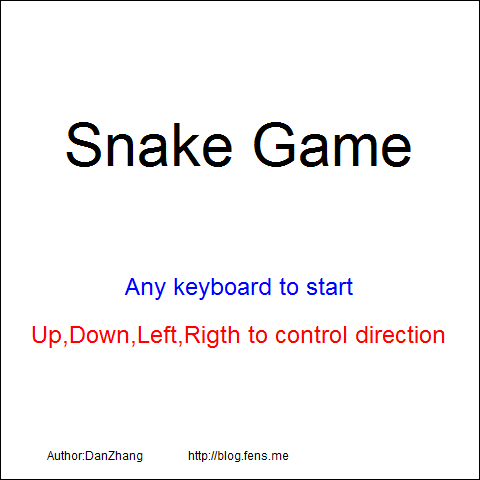This entry was posted in R语言实践, 游戏

Article Rating
Subscribe
Notify ofThis site uses Akismet to reduce spam. Learn how your comment data is processed.

Inline Feedbacks[…] R语言游戏之旅 贪食蛇入门 […][…] R语言游戏之旅 贪食蛇入门, R语言游戏框架设计, R语言游戏之旅 […]Win下，可以从github安装这个包。
https://github.com/bsspirit/gridgame

library(devtools)
install_github(“gridgame”,”bsspirit”)
snake() # start snake game.
g2048() # start 2048 game.Error in setGraphicsEventEnv(which, as.environment(list(…))) :
this graphics device does not support event handlingRstudio有一个GUI的设置，他重置了grDevices库。R的3.2.2版本以后，R就就可以了，等Rstudio更新那个配置就行了。

12
0